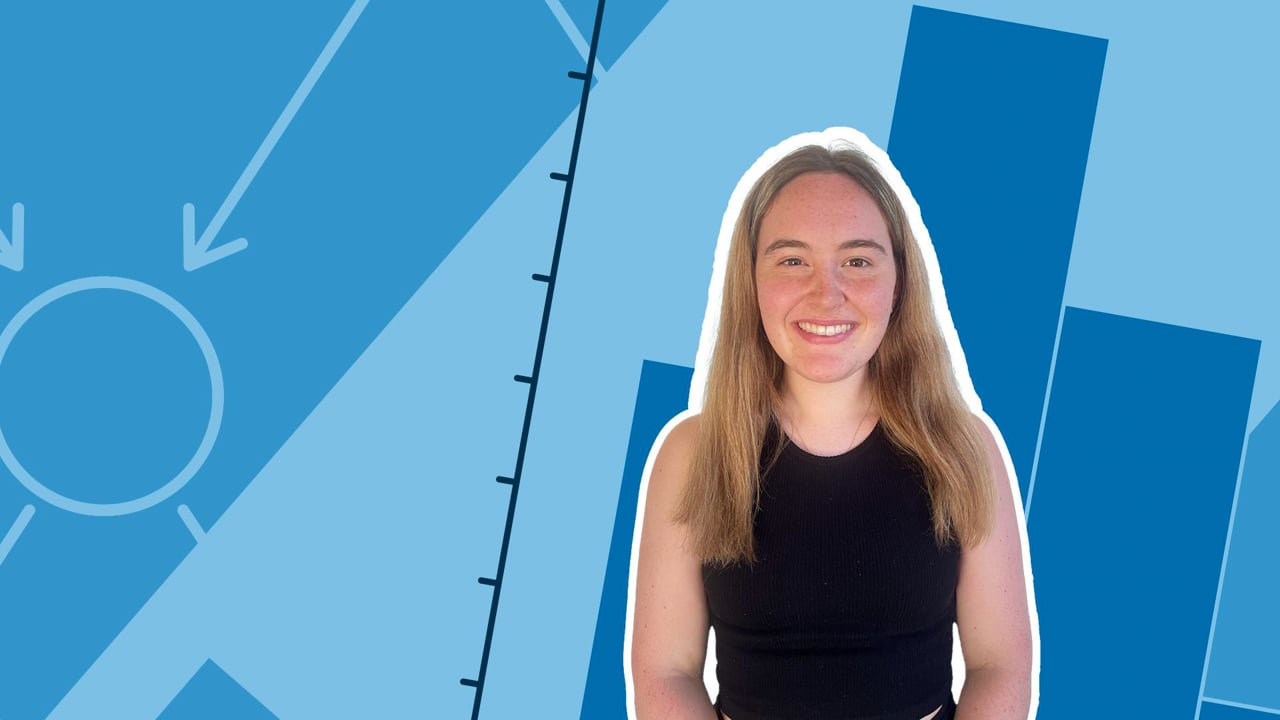Chapter OverviewMaths

Number and place value

Multiplication and division

Fractions

Measurement

Geometry - properties of shapes

Geometry - position and direction

Statistics

Maths

# Discrete and continuous data0%

Summary

# Discrete and continuous data

## ​​In a nutshell

There are two different kinds of data: discrete and continuous. Different diagrams are used depending on the data type.

## Different kinds of data

#### EXAMPLE

Discrete

Whole number data that is counted, not measured

The number of books on each shelf in a library

Continuous

Measurable data that could take any value

The height of students in class

### Displaying different data types

Deciding the type of numerical data you have allows you to decide which chart, graph or diagram to use.

• For discrete data the best type of diagram to use is a bar chart.
• For continuous data the best type of diagram to use is a line graph.

FAQs

• Question: What is an example of continuous data?

Answer: The heights of students in a class.

• Question: What is an example of discrete data?

Answer: The number of books on each shelf in a library

• Question: What is continuous data?

Answer: Measurable data that could take any value

• Question: What is discrete data?

Answer: Discrete data is whole number data that is counted, not measured.

Theory

Exercises